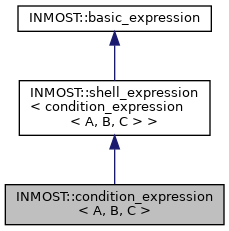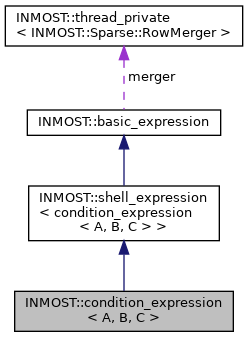INMOST A toolkit for distributed mathematical modeling
INMOST::condition_expression< A, B, C > Class Template Reference
Inheritance diagram for INMOST::condition_expression< A, B, C >:[legend]
Collaboration diagram for INMOST::condition_expression< A, B, C >:[legend]

## Public Member Functions

condition_expression (const shell_expression< A > &pcond, const shell_expression< B > &pleft, const shell_expression< C > &pright)

condition_expression (const condition_expression &other)

condition_expression (const condition_expression &other, const A &pcond, const B &pleft, const C &pright)

__INLINE INMOST_DATA_REAL_TYPE GetValue () const

__INLINE void GetJacobian (INMOST_DATA_REAL_TYPE mult, Sparse::RowMerger &r) const

__INLINE void GetJacobian (INMOST_DATA_REAL_TYPE mult, Sparse::Row &r) const

__INLINE void GetHessian (INMOST_DATA_REAL_TYPE multJ, Sparse::Row &J, INMOST_DATA_REAL_TYPE multH, Sparse::HessianRow &H) const

__INLINE INMOST_DATA_ENUM_TYPE GetCount () constPublic Member Functions inherited from INMOST::shell_expression< condition_expression< A, B, C > >
operator condition_expression< A, B, C > & ()

operator const condition_expression< A, B, C > & () constStatic Protected Attributes inherited from INMOST::basic_expression